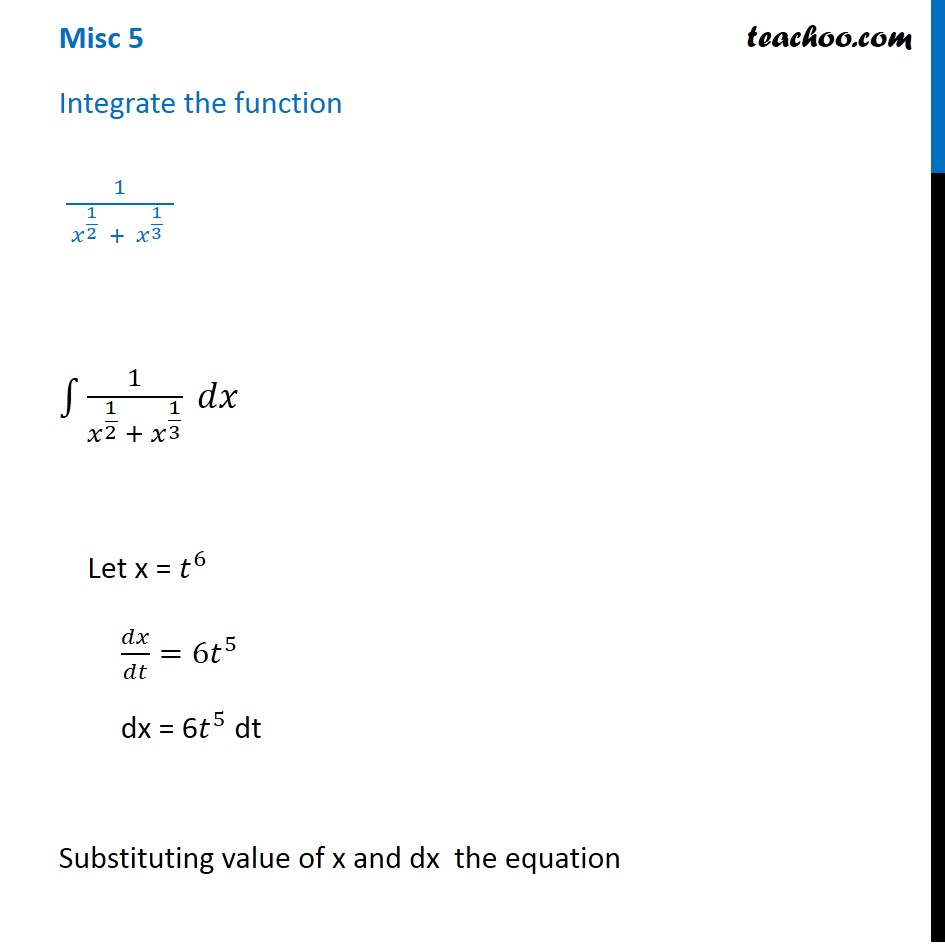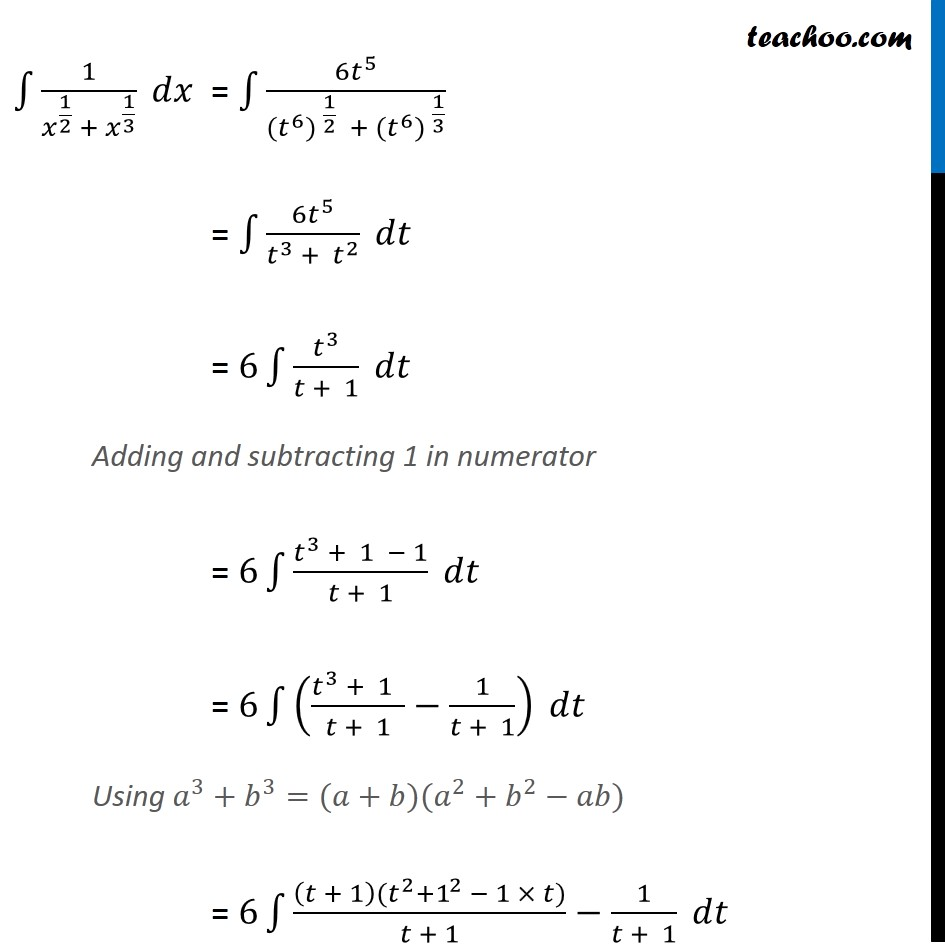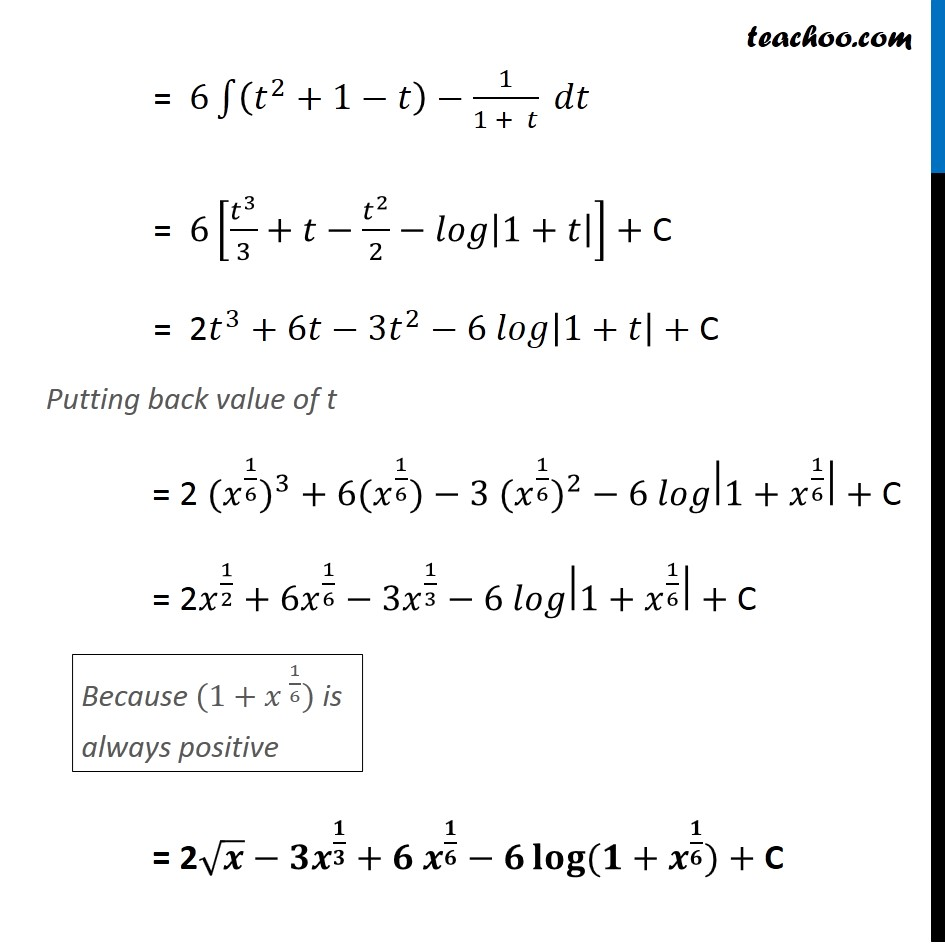Miscellaneous

Chapter 7 Class 12 Integrals
Serial order wiseLearn in your speed, with individual attention - Teachoo Maths 1-on-1 Class

### Transcript

Misc 5 Integrate the function 1/( 𝑥^(1/2) + 𝑥^(1/3) ) ∫1▒1/(𝑥^(1/2) + 𝑥^(1/3) ) 𝑑𝑥 Let x = 𝑡^6 𝑑𝑥/𝑑𝑡=6𝑡^5 dx = 6𝑡^5 dt Substituting value of x and dx the equation ∫1▒1/(𝑥^(1/2) + 𝑥^(1/3) ) 𝑑𝑥 = ∫1▒〖6𝑡〗^5/(〖〖(𝑡〗^6) 〗^(1/2) +〖〖 (𝑡〗^6) 〗^(1/3) ) = ∫1▒〖6𝑡〗^5/(𝑡^3 + 𝑡^2 ) 𝑑𝑡 = 6∫1▒𝑡^3/(𝑡 + 1) 𝑑𝑡 Adding and subtracting 1 in numerator = 6∫1▒(𝑡^3 + 1 − 1)/(𝑡 + 1) 𝑑𝑡 = 6∫1▒((𝑡^3 + 1 )/(𝑡 + 1)−1/(𝑡 + 1)) 𝑑𝑡 Using 𝑎^3+𝑏^3=(𝑎+𝑏)(𝑎^2+𝑏^2−𝑎𝑏) = 6∫1▒((𝑡 + 1)(𝑡^2+1^2 − 1 × 𝑡))/(𝑡 + 1)−1/(𝑡 + 1) 𝑑𝑡 = 6∫1▒〖(𝑡^2+1−𝑡)−1/(1 + 𝑡)〗 𝑑𝑡 = 6[𝑡^3/3+𝑡−𝑡^2/2−𝑙𝑜𝑔|1+𝑡|]+ C = 2𝑡^3+6𝑡−〖3𝑡〗^2−6 𝑙𝑜𝑔|1+𝑡|+ C Putting back value of t = 2 〖〖(𝑥〗^(1/6))〗^3+6〖(𝑥〗^(1/6))−3 〖〖(𝑥〗^(1/6))〗^2−6 𝑙𝑜𝑔|1+𝑥^(1/6) |+ C = 2𝑥^(1/2)+6𝑥^(1/6)−3𝑥^(1/3)−6 𝑙𝑜𝑔|1+𝑥^(1/6) |+ C = 2√𝒙−𝟑𝒙^(𝟏/𝟑)+〖𝟔 𝒙〗^(𝟏/𝟔)−𝟔 𝐥𝐨𝐠⁡〖(𝟏+𝒙^(𝟏/𝟔))〗+ C Because (1+〖𝑥 〗^(1/6)) is always positive